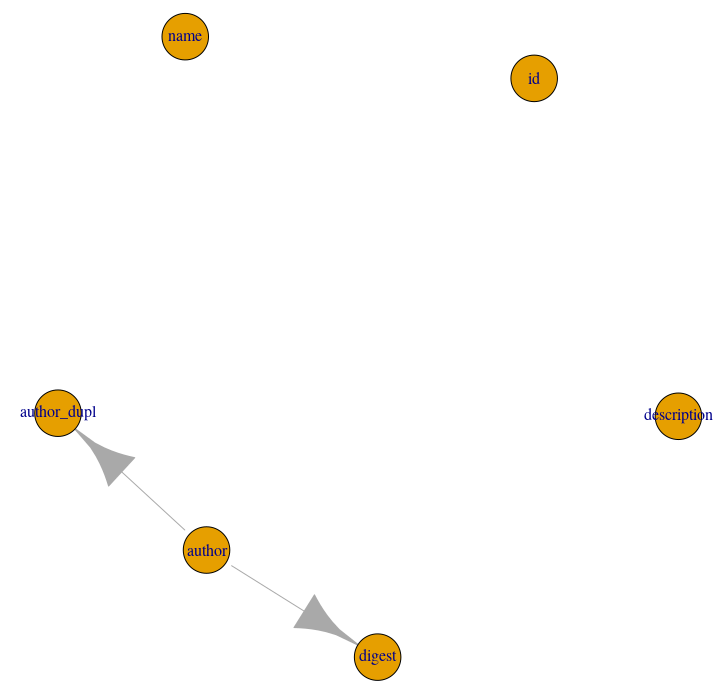# DataFakeR workflow

#### 2023-02-10

The main goal of DataFakeR package is to simulate fake data based on table(s) configuration.

### Creating table schema

The configuration file describing schema can be automatically created based on database configuration, R named list of tables and/or customized manually (see schema structure).

When the schema should be dumped from database simply run:

schema <- schema_source(
source = <db-connection-object>,
schema_name = <target-schema-name>,
file = <target-schema-configuration-file>
)

If you want to create dump from list of tables run:

schema <- schema_source(
source = <named-list-of-tables>,
schema_name = <target-schema-name>,
file = <target-schema-configuration-file>
)

The function will read necessary schema information and save it in file yaml file. As a result the new R6 object will be returned storing all the necessary information about the datasets, that allow the package to perform simulation step.

Note: You may customize data sourcing options using schema_source’s faker_opts parameter. See dump configuration.

If you already have defined yaml configuration file, you may also use schema_source to read the schema object. Just use the function providing schema file path as a source parameter:

schema <- schema_source(
source = <source-schema-configuration-file>
)

Note: You can see both schema sourcing and simulation progress by setting options("dfkr_verbose" = TRUE).

### Simulating data

In order to simulate the data, pass schema object to schema_simulate function:

schema <- schema_simulate(schema)

The simulation process is highly configurable. For more details see simulation options.

### Accessing simulated data

The simulated tables can be accessed with using schema_get_table function:

table_a <- schema_get_table(schema, <table-name>)

## The other schema methods

### Display table dependencies

In order to perform tables simulation and preserve its original structure DataFakeR detects dependencies between tables and columns. Such dependencies sets the order of tables and columns simulation. For example having table_a$column_a as a foreign key for table_b$column_b, table_b will be simulated before table_a.

Table dependencies are defined by foreign keys definition, whereas inner-table column dependencies consider multiple parameters defined in yaml configuration. Such cases are:

• the column is grouped by another one,
• the column is bounded by equality constraint with another one,
• the column is defined by formula in which the other column was used.

To check tables dependencies use:

schema_plot_deps(schema)

To check column dependencies for selected table use:

schema_plot_deps(schema, table_name = <table-name>)

Example output:### Updating schema object

While trying to simulate the target data you may want to improve the currently defined configuration file. If you want to update the object using new version of the file (and new simulation options) without the need to source it from scratch just use:

schema <- schema_update_source(
schema,
file = <new-version-source-file>,
faker_opts = <new-simulation-options>
)